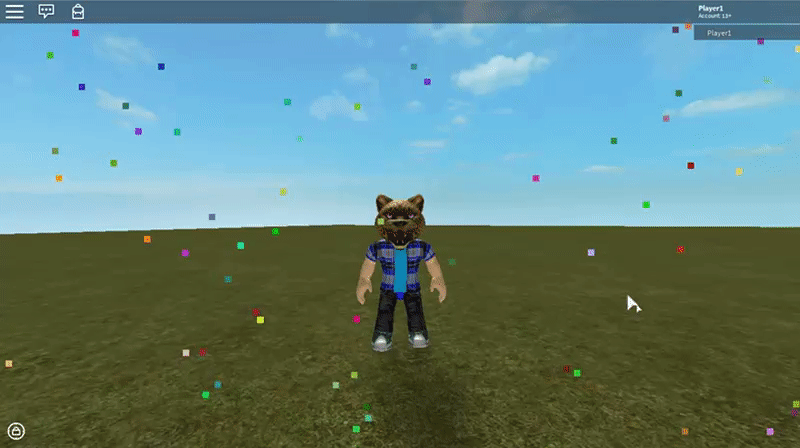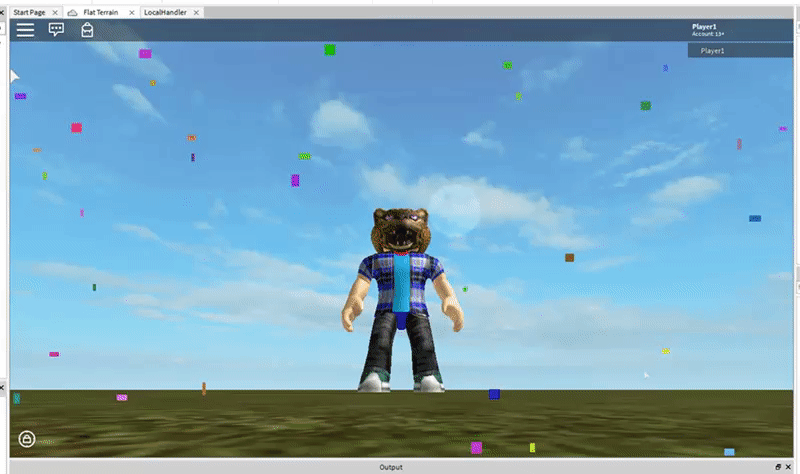# Bouncing Frame GUIs

• I made thisHere's the link to the model. Just put this script in ReplicatedFirst, and click the screen while playing with a character to make GUIsHere's the bulk of the code:

``````--// GUI Stuff
local frame     = script.Parent
local size      = frame.Size
size            = Vector2.new(size.X.Offset, size.Y.Offset)
local screenGui = frame.Parent

--// Static Vars
local screenSize = screenGui.AbsoluteSize
screenGui.Changed:Connect(function(property)
if(property=="AbsoluteSize")then
screenSize = screenGui.AbsoluteSize
end
end)
local direction = Vector2.new(({1,-2})[math.random(2)],({1,-2})[math.random(2)])

--// Public Vars
local speed = 5

speed = speed+math.random()
game:GetService("RunService").RenderStepped:Connect(function() -- use bindtorenderstepped later
local pos        = frame.Position
if(screenSize.X<=pos.X.Offset+size.X)then
direction = Vector2.new(-1, direction.Y)
elseif(pos.X.Offset<=0)then
direction = Vector2.new(1, direction.Y)
end
if(screenSize.Y<=pos.Y.Offset+size.Y)then
direction = Vector2.new(direction.X, -1)
elseif(pos.Y.Offset<=0)then
direction = Vector2.new(direction.X, 1)
end
frame.Position = frame.Position+UDim2.new(0,direction.X*speed,0,direction.Y*speed)
end)
``````

• This will be a great substition for the Roblox particle effect!

• @shabbs15 Maybe, I guess, lol.

Not sure if this would be less laggy.

It just looks neat yay!Looks like your connection to Scripting Helpers was lost, please wait while we try to reconnect.